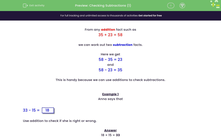# Use Addition to Check Subtractions

In this worksheet, students will use additions to check subtractions.Key stage:  KS 2

Curriculum topic:   Number: Addition and Subtraction

Curriculum subtopic:   Estimate and Check Answers

Popular topics:   Subtraction worksheets, Addition worksheets

Difficulty level:#### Worksheet Overview

From any addition fact such as

35 + 23 = 58

we can work out two subtraction facts.

Here we get

58 - 35 = 23

and

58 - 23 = 35

This is handy because we can use additions to check subtractions.Example 1

Anna says that

33 - 15 = 18

Use addition to check if she is right or wrong.

18 + 15 = 33

So Anna is right.

Example 2

Ben says that

54 - 15 = 31

Use addition to check if he is right or wrong.

31 + 15 = 46 not 54

So Ben is wrong.

Are you happy to try some questions on this now?### What is EdPlace?

We're your National Curriculum aligned online education content provider helping each child succeed in English, maths and science from year 1 to GCSE. With an EdPlace account you’ll be able to track and measure progress, helping each child achieve their best. We build confidence and attainment by personalising each child’s learning at a level that suits them.

Get started#### Popular Maths topics

••••